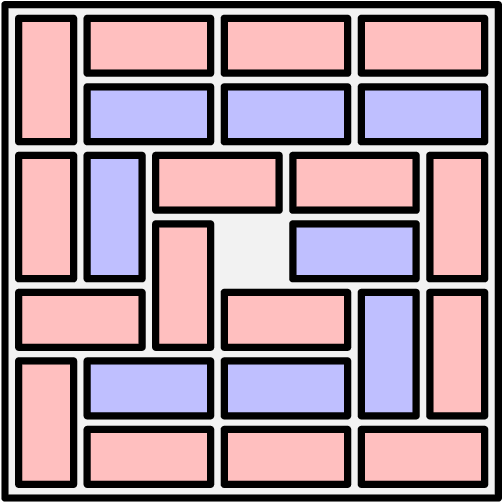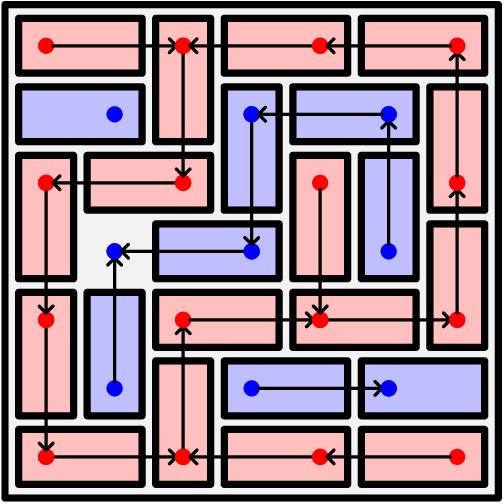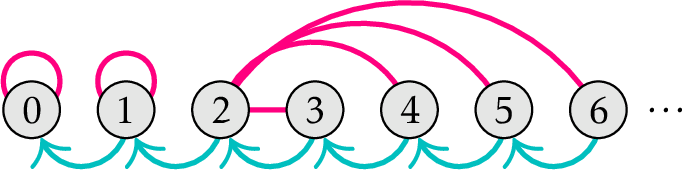lvshu

V1

2023/03/26阅读：61主题：自定义主题1

# 2023 USAMO

### 绿树教育中心独家解析

，则有 ，则可得到 。再由于 ，则 ，故 ，即证得

### 绿树教育中心独家解析

If , then

implies .

By and , we have

implying by injectivity.

For any , note that summing

gives

The above equation uniquely determines in terms of , , , and it is clear , , satisfies the equation, so are collinear.

### 绿树教育中心独家解析

The answer is . Call a cell blue if its row index and column index are both even, and call a cell red if its row index and column index are both odd. Additionally, color each domino with the color of the colored cell it covers.

#### Constructions

One possible construction for involves positioning the dominoes covering red cells in a snake-like fashion. An example construction for is shown below.One possible construction for involves positioning the dominoes covering blue cells in a snake-like fashion, blocking the snake's path with a red domino and an empty square, and filling the rest of the grid with red dominoes. An example construction for and is given below.#### Proof that no other work

Let be the directed graph whose vertex set is the red and blue cells, and whose directed edges are drawn from to the cell the domino covering points to, if it exists.Let denote the uncovered cell. Observe the following: (i) By a checkerboard coloring argument, must be a vertex of . (ii) Sliding a domino does not change the location of 's edges; it only reverses the direction of an arrow. (iii) The number of cells in any cycle's interior is odd, so if contains a cycle, then is inside the cycle. (iv) The connected component of containing is a tree because it cannot contain any cycles, by (iii). (v) Given the nondirected edges of , specifying the uncovered cell is enough to recover the domino configuration, by (iv). (vi) Hence, is the number of vertices in the connected component of containing . Because arrows only connect cells of the same color, is at most the number of red cells, which is . Therefore, it suffices to prove that . This follows from the following lemma:

Lemma wrote: If the connected component containing has more than vertices, then it contains every red vertex.

Proof. If the connected component containing has more than vertices, then must be red because there are only blue vertices. Additionally, at least one vertex in the connected component containing must border the edge of the grid, so without loss of generality assume borders the edge of the grid by sliding some of the dominoes.

Now, starting from the red vertex and following the arrows must eventually lead to or lead to a cycle. By (iii), it is impossible to get into a cycle because is on the edge. Thus, every red vertex is connected to , as desired.

Therefore, implies that equals the number of red cells, which is . This gives the solution set , as desired.

### 绿树教育中心独家解析

Rewrite each number as its 2-adic valuation; then the problem becomes equivalent to moving tokens on the graph below, where Alice's moves are denoted by magenta edges, and Bob's moves --- cyan. (The picture shows an example for .)If Alice has any tokens to the right of or at , then she wins by stalling until Bob moves a token there, and then ''shooting'' that token outwards so that Bob has to move it again. Otherwise it's clear that Alice is basically useless, and Bob is playing with a ticking fuse until the game is over.

### 绿树教育中心独家解析

If is prime, note that all arithmetic progressions of length either are all the same or contain every residue . Note that if one row has all the same residues, then no row can contain all the residues, since there are only numbers with the same residue less than . Hence, if one row has all the same residues, then all the rows do. In this case, we can permute the rows such that the columns are (in some order)

for all . Otherwise, all rows have all different residues. In this case, sort all the rows, and the columns are (in some order) for all . Hence, the statement is true for all prime . If is composite, write and consider the construction

Suppose for the sake of contradiction that you can rearrange this table to be column-valid. Consider the arithmetic progression in the column containing . Let it's common difference be . Note that for the th term to not be in the first row, we require

Since the th term is at most , we have

Note that because otherwise the second term would be in the first row. Hence, . However, we now have that the th term is

but then no terms can be from the last row. Hence, no column-valid rearrangement exists.

### 绿树教育中心独家解析

ENDV1